International
Tables for
Crystallography
Volume D
Physical properties of crystals
Edited by A. Authier

International Tables for Crystallography (2006). Vol. D, ch. 1.1, pp. 14-16

## Section 1.1.4.6. Symmetry of tensors imposed by the crystalline medium

A. Authiera*

aInstitut de Minéralogie et de la Physique des Milieux Condensés, Bâtiment 7, 140 rue de Lourmel, 75015 Paris, France
Correspondence e-mail: aauthier@wanadoo.fr

#### 1.1.4.6. Symmetry of tensors imposed by the crystalline medium

| top | pdf |

Many papers have been devoted to the derivation of the invariant components of physical property tensors under the influence of the symmetry elements of the crystallographic point groups: see, for instance, Fumi (1951, 1952a,b,c, 1987), Fumi & Ripamonti (1980a,b), Nowick (1995), Nye (1957, 1985), Sands (1995), Sirotin & Shaskol'skaya (1982), and Wooster (1973). There are three main methods for this derivation: the matrix method (described in Section 1.1.4.6.1), the direct inspection method (described in Section 1.1.4.6.3) and the group-theoretical method (described in Section 1.2.4and used in the accompanying software, see Section 1.2.7.4).

#### 1.1.4.6.1. Matrix method – application of Neumann's principle

| top | pdf |

An operation of symmetry turns back the crystalline edifice on itself; it allows the physical properties of the crystal and the tensors representing them to be invariant. An operation of symmetry is equivalent to a change of coordinate system. In a change of system, a tensor becomes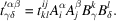If A represents a symmetry operation, it is a unitary matrix: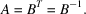Since the tensor is invariant under the action of the symmetry operator A, one has, according to Neumann's principle,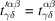and, therefore,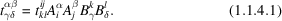There are therefore a certain number of linear relations between the components of the tensor and the number of independent components is reduced. If there are p components and q relations between the components, there areindependent components. This number is independent of the system of axes. When applied to each of the 32 point groups, this reduction enables one to find the form of the tensor in each case. It depends on the rank of the tensor. In the present chapter, the reduction will be derived for tensors up to the fourth rank and for all crystallographic groups as well as for the isotropic groups. An orthonormal frame will be assumed in all cases, so that co- and contravariance will not be apparent and the positions of indices as subscripts or superscripts will not be meaningful. The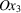axis will be chosen parallel to the threefold, fourfold or sixfold axis in the trigonal, tetragonal and hexagonal systems. The accompanying software to the present volume enables the reduction for tensors of any rank to be derived.

| top | pdf |

#### 1.1.4.6.2.1. Introduction

| top | pdf |

If one takes as the system of axes the eigenvectors of the operator A, the matrix is written in the form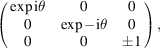where θ is the rotation angle,is taken parallel to the rotation axis and coefficientis equal to +1 or −1 depending on whether the rotation axis is direct or inverse (proper or improper operator).

The equations (1.1.4.1)can then be simplified and reduce to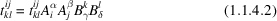(without any summation).

If the product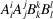(without summation) is equal to unity, equation (1.1.4.2)is trivial and there is significance in the component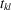. On the contrary, if it is different from 1, the only solution for (1.1.4.2)is that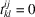. One then finds immediately that certain components of the tensor are zero and that others are unchanged.

#### 1.1.4.6.2.2. Case of a centre of symmetry

| top | pdf |

All the diagonal components are in this case equal to −1. One thus has:

 (i) Tensors of even rank,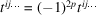. The components are not affected by the presence of the centre of symmetry. The reduction of tensors of even rank is therefore the same in a centred group and in its noncentred subgroups, that is in any of the 11 Laue classes: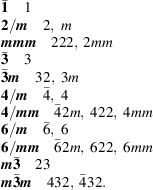If a tensor is invariant with respect to two elements of symmetry, it is invariant with respect to their product. It is then sufficient to make the reduction for the generating elements of the group and (since this concerns a tensor of even rank) for the 11 Laue classes. (ii) Tensors of odd rank,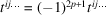. All the components are equal to zero. The physical properties represented by tensors of rank 3, such as piezoelectricity, piezomagnetism, nonlinear optics, for instance, will therefore not be present in a centrosymmetric crystal.

#### 1.1.4.6.2.3. General case

| top | pdf |

By replacing the matrix coefficients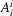by their expression, (1.1.4.2)becomes, for a proper rotation,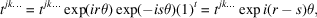where r is the number of indices equal to 1, s is the number of indices equal to 2, t is the number of indices equal to 3 and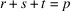is the rank of the tensor. The component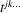is not affected by the symmetry operation if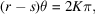where K is an integer, and is equal to zero if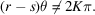The angle of rotation θ can be put into the form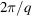, where q is the order of the axis. The condition for the component not to be zero is then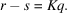The condition is fulfilled differently depending on the rank of the tensor, p, and the order of the axis, q. Indeed, we have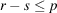and,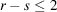: the result of the reduction will be the same for any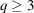;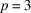,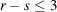: the result of the reduction will be the same for any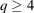;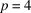,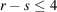: the result of the reduction will be the same for any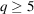.

It follows that:

 (i) for tensors of rank 2, the reduction will be the same for trigonal (threefold axis), tetragonal (fourfold axis) and hexagonal (sixfold axis) groups; (ii) for tensors of rank 3, the reduction will be the same for tetragonal and hexagonal groups; (iii) for tensors of rank 4, the reduction will be different for trigonal, tetragonal and hexagonal groups.

The inconvenience of the diagonalization method is that the vectors and eigenvalues are, in general, complex, so in practice one uses another method. For instance, we may note that equation (1.1.4.1)can be written in the case ofby associating with the tensor amatrix T: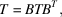where B is the symmetry operation. Through identification of homologous coefficients in matrices T and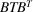, one obtains relations between components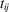that enable the determination of the independent components.

#### 1.1.4.6.3. The method of direct inspection

| top | pdf |

The method of direct inspection', due to Fumi (1952a,b, 1987), is very simple. It is based on the fundamental properties of tensors; the components transform under a change of basis like a product of vector components (Section 1.1.3.2).

#### Examples

 (1) Let us consider a tensor of rank 3 invariant with respect to a twofold axis parallel to. The matrix representing this operator is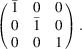The component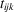behaves under a change of axes like the product of the components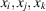. The componentsof a vector become, respectively,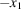,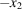,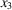. To simplify the notation, we shall denote the components of the tensor simply by ijk. If, amongst the indices i, j and k, there is an even number (including the number zero) of indices that are equal to 3, the product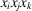will become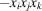under the rotation. As the component ijk' remains invariant and is also equal to its opposite, it must be zero. 14 components will thus be equal to zero: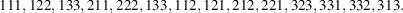(2) Let us now consider that the same tensor of rank 3 is invariant with respect to a fourfold axis parallel to. The matrix representing this operator and its action on a vector of coordinatesis given by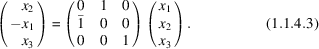Coordinate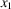becomes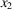,becomesandbecomes. Component ijk transforms like product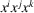according to the rule given above. Since the twofold axis parallel tois a subgroup of the fourfold axis, we can start from the corresponding reduction. We find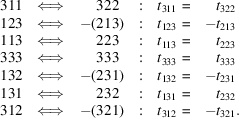All the other components are equal to zero.

It is not possible to apply the method of direct inspection for point group 3. One must in this case use the matrix method described in Section 1.1.4.6.2; once this result is assumed, the method can be applied to all other point groups.

### References

Fumi, F. G. (1951). Third-order elastic coefficients of crystals. Phys. Rev. 83, 1274–1275.
Fumi, F. G. (1952a). Physical properties of crystals: the direct inspection method. Acta Cryst. 5, 44–48.
Fumi, F. G. (1952b). The direct-inspection method in systems with a principal axis of symmetry. Acta Cryst. 5, 691–694.
Fumi, F. G. (1952c). Third-order elastic coefficients in trigonal and hexagonal crystals. Phys. Rev. 86, 561.
Fumi, F. G. (1987). Tables for the third-order elastic tensors in crystals. Acta Cryst. A43, 587–588.
Fumi, F. G. & Ripamonti, C. (1980a). Tensor properties and rotational symmetry of crystals. I. A new method for group 3(3z) and its application to general tensors up to rank 8. Acta Cryst. A36, 535–551.
Fumi, F. G. & Ripamonti, C. (1980b). Tensor properties and rotational symmetry of crystals. II. Groups with 1-, 2- and 4-fold principal symmetry and trigonal and hexagonal groups different from group 3. Acta Cryst. A36, 551–558.
Nowick, A. S. (1995). Crystal properties via group theory. Cambridge University Press.
Nye, J. F. (1957). Physical properties of crystals, 1st ed. Oxford: Clarendon Press.
Nye, J. F. (1985). Physical properties of cystals, revised ed. Oxford University Press.
Sands, D. E. (1995). Vectors and tensors in crystallography. New York: Dover.
Sirotin, Y. I. & Shaskol'skaya, M. P. (1982). Fundamentals of crystal physics. Moscow: Mir.
Wooster, W. A. (1973). Tensors and group theory for the physical properties of crystals. Oxford: Clarendon Press.